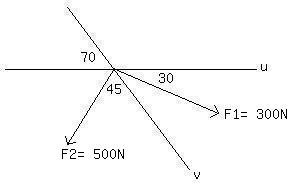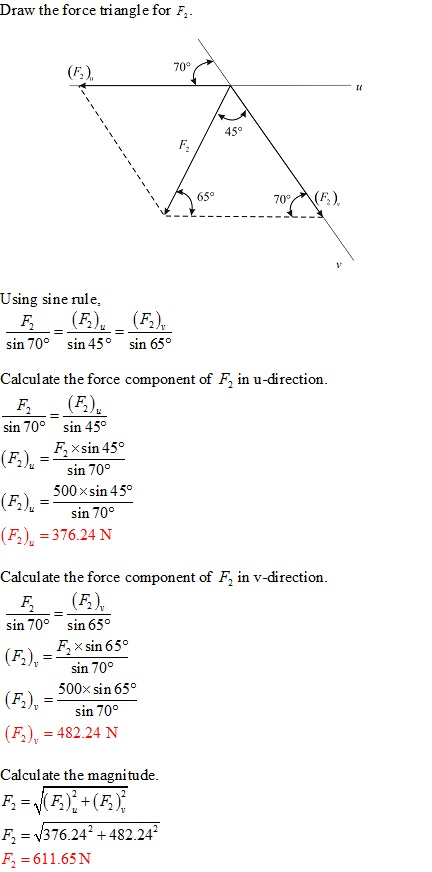# Resolve the force F2 into components acting along the u and v axes and determineLipossig 2021-02-11 Answered

Resolve the force F2 into components acting along the u and v axes and determine the magnitudes of the components.You can still ask an expert for help

• Questions are typically answered in as fast as 30 minutes

Solve your problem for the price of one coffee

• Math expert for every subject
• Pay only if we can solve itNeelam Wainwright.

###### Not exactly what you’re looking for?Jeffrey Jordon

By sine rule:

$\frac{{F}_{u}}{\mathrm{sin}{45}^{\circ }}=\frac{{F}_{v}}{\mathrm{sin}{30}^{\circ }}=\frac{{F}_{z}}{\mathrm{sin}{75}^{\circ }}$

$⇒{F}_{u}=\frac{{F}_{z}}{\mathrm{sin}{75}^{\circ }}×\mathrm{sin}{75}^{\circ }$

and ${F}_{v}=\frac{{F}_{z}}{\mathrm{sin}{75}^{\circ }}×\mathrm{sin}{30}^{\circ }=\frac{6}{\mathrm{sin}{75}^{\circ }}×\mathrm{sin}{30}^{\circ }$